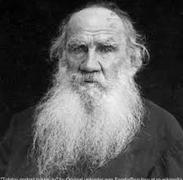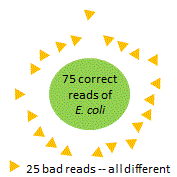If most bases are good, most unique sequences are bad, because

Dominant OTUs

Spurious OTUs in mock vs. real samples

Suppose our reads have length 250nt, and with stringent quality filtering, all the bases have Q40 (in practice, this is unrealistic). Q40 means P_error = 0.001 = 1/1000, and on average one letter will be wrong out of every thousand. That's four reads of length 250nt, so 75% of reads will be correct and 25% of reads will have a bad base.

Now suppose we make 100 reads of the same template sequence, say for E. coli. We will get 75 correct reads, all with the same unique sequence. We will also get 25 bad reads, each of which will probably have a different unique sequence because errors tend to be random so are only rarely reproduced.

This gives a total of 26 unique sequences, 25 of which are bad. Only 1/26 = 4% of the unique sequences are correct! Thus, in this scenario,

99.9% correct bases = 75% correct reads = 96% incorrect unique sequences.

This result depends on the read depth. If we made 12 reads per template, then we would get 1 correct sequence and 3 bad sequences, so 3/4 = 75% of the uniques would be bad. If we made 1,000 reads per template, then 249/250 = 99.6% of uniques would be bad.

So we should ask: what is the average read depth in practice? That depends on the diversity of the community and the number of reads. Suppose we have 20 samples, and the average number of reads per species per sample is 5. This gives 100 reads per species when all samples are combined, and hence 96% bad uniques as above (assuming Q40 for all bases and no PCR errors). If there are 5,000 reads per sample, which is a typical number these days, then we get an average read depth of 5 reads / species if there are 1,000 species in the reads, which I believe is typical or perhaps at the high end, as a rough ballpark.

If the rate of bad uniques increases with read depth, isn't this an argument for clustering or denoising each sample individually? No, because combining samples improves detection of chimeras and bad reads.

In real life, things can be much worse for several reasons. If we required every base call to be Q40 we would lose all our reads, so we have to accept less stringent filtering. Also, quality filtering doesn't catch chimeras and other PCR errors (substitutions and indels due to polymerase copying mistakes). So in practice, we should expect that a large majority of unique sequences will have errors.

Most of bad uniques have one only wrong base, and these are relatively harmless unless the correct sequence is close to the boundary of an OTU, in which case the error could induce a spurious OTU. However, errors correlate and fluctuate for a variety of reasons which often cause a small but significant minority of bad reads to have >3% incorrect bases. The average error rate can be misleading -- what really matters is the shape of the distribution, and in particular how many reads have larger number of errors. These reads are rare as a fraction of the total number of reads, but nevertheless can create many spurious OTUs unless precautions are taken such as discarding singletons.